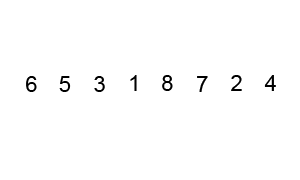# Bubble Sort• Sinking sort
• Similar to selection sort with a different scheme for finding min value
• Compares successive elements, swapping whenever the bottom element is smaller than the one above it
• Smallest element "bubbles up" to the front of the array
• Each iteration puts the smallest unsorted element in its correct place
• Inefficient but can quit early if partially sorted

## Complexity

Average TimeSpace
$O(n^2)$$1$

## Process

• Repeatedly step through list
• Compare each pair of adjacent elements --> swap if they are in the wrong order
• Pass through the list until no swaps are needed

## Implementations

### JavaScript

function bubbleSort(array) {
// Keep track if we swap an element
let swapped = false;

// Start looping through the entire array
// i keeps track of how many elements are sorted
for (let i = 1; i < array.length; i++) {
// Each new element resets the swap tracker
swapped = false;

// Compare element with all remaining elements in array
for (let j = 0; j < array.length - i; j++) {
// If a smaller element is found, swap in place
if (array[j + 1] < array[j]) {
[array[j], array[j + 1]] = [array[j + 1], array[j]];

// Mark as swapped
swapped = true;
}
}

// Return early if nothing is swapped
if (!swapped) {
return array;
}
}

return array;
}